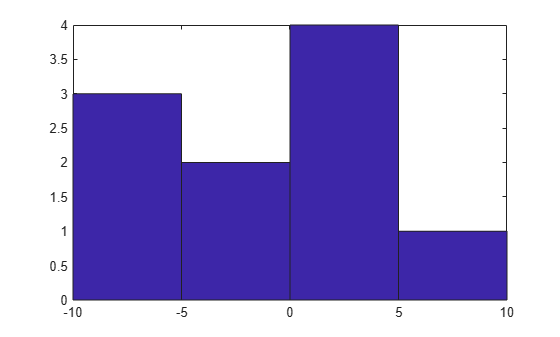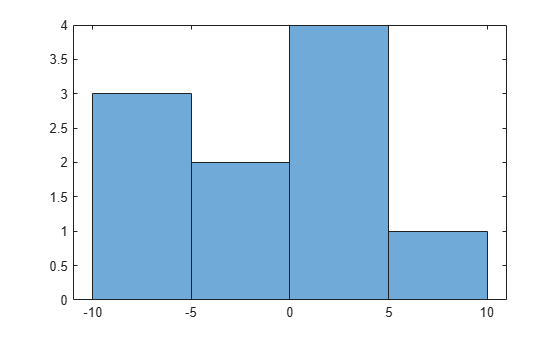## Replace Discouraged Instances of hist and histc

### Old Histogram Functions (`hist`, `histc`)

Earlier versions of MATLAB® use the `hist` and `histc` functions as the primary way to create histograms and calculate histogram bin counts. These functions, while good for some general purposes, have limited overall capabilities. The use of `hist` and `histc` in new code is discouraged for these reasons (among others):

• After using `hist` to create a histogram, modifying properties of the histogram is difficult and requires recomputing the entire histogram.

• The default behavior of `hist` is to use 10 bins, which is not suitable for many data sets.

• Plotting a normalized histogram requires manual computations.

• `hist` and `histc` do not have consistent behavior.

### Recommended Histogram Functions

The `histogram`, `histcounts`, and `discretize` functions dramatically advance the capabilities of histogram creation and calculation in MATLAB, while still promoting consistency and ease of use. `histogram`, `histcounts`, and `discretize` are the recommended histogram creation and computation functions for new code.

Of particular note are the following changes, which stand as improvements over `hist` and `histc`:

• `histogram` can return a histogram object. You can use the object to modify properties of the histogram.

• Both `histogram` and `histcounts` have automatic binning and normalization capabilities, with several common options built-in.

• `histcounts` is the primary calculation function for `histogram`. The result is that the functions have consistent behavior.

• `discretize` provides additional options and flexibility for determining the bin placement of each element.

Despite the aforementioned improvements, there are several important differences between the old and now recommended functions, which might require updating your code. The tables summarize the differences between the functions and provide suggestions for updating code.

Code Updates for `hist`

DifferenceOld behavior with `hist`New behavior with `histogram`

Input matrices

`hist` creates a histogram for each column of an input matrix and plots the histograms side-by-side in the same figure.

```A = randn(100,2); hist(A)```

`histogram` treats the input matrix as a single tall vector and creates a single histogram. To plot multiple histograms, create a different histogram object for each column of data. Use the `hold on` command to plot the histograms in the same figure.

```A = randn(100,2); h1 = histogram(A(:,1),10) edges = h1.BinEdges; hold on h2 = histogram(A(:,2),edges) ```

The above code example uses the same bin edges for each histogram, but in some cases it is better to set the `BinWidth` of each histogram to be the same instead. Also, for display purposes, it might be helpful to set the `FaceAlpha` property of each histogram, as this affects the transparency of overlapping bars.

Bin specification

`hist` accepts the bin centers as a second input.

`histogram` accepts the bin edges as a second input.

To convert bin centers into bin edges for use with `histogram`, see Convert Bin Centers to Bin Edges.

Note

In cases where the bin centers used with `hist` are integers, such as `hist(A,-3:3)`, use the new built-in binning method of `histogram` for integers.

`histogram(A,'BinLimits',[-3,3],'BinMethod','integers')`

Output arguments

`hist` returns the bin counts as an output argument, and optionally can return the bin centers as a second output argument.

```A = randn(100,1); [N, Centers] = hist(A)```

`histogram` returns a histogram object as an output argument. The object contains many properties of interest (bin counts, bin edges, and so on). You can modify aspects of the histogram by changing its property values. For more information, see `histogram`.

```A = randn(100,1); h = histogram(A); N = h.Values Edges = h.BinEdges```

Note

To calculate bin counts (without plotting a histogram), replace `[N, Centers] = hist(A)` with ```[N,edges] = histcounts(A,nbins)```.

Default number of bins

`hist` uses 10 bins by default.

Both `histogram` and `histcounts` use an automatic binning algorithm by default. The number of bins is determined by the size and spread of the input data.

```A = randn(100,1); histogram(A) histcounts(A)```

Bin limits

`hist` uses the minimum and maximum finite data values to determine the left and right edges of the first and last bar in the plot. `-Inf` and `Inf` are included in the first and last bin, respectively.

If `BinLimits` is not set, then `histogram` uses rational bin limits based on, but not exactly equal to, the minimum and maximum finite data values. `histogram` ignores `Inf` values unless one of the bin edges explicitly specifies `Inf` or `-Inf` as a bin edge.

To reproduce the results of `hist(A)` for finite data (no `Inf` values), use 10 bins and explicitly set `BinLimits` to the minimum and maximum data values.

```A = randi(5,100,1); histogram(A,10,'BinLimits',[min(A) max(A)])```

Code Updates for `histc`

DifferenceOld behavior with `histc`New behavior with `histcounts`
Input matrices

`histc` calculates the bin counts for each column of input data. For an input matrix of size `m`-by-`n`, `histc` returns a matrix of bin counts of size `length(edges)`-by-`n`.

```A = randn(100,10); edges = -4:4; N = histc(A,edges)```

`histcounts` treats the input matrix as a single tall vector and calculates the bin counts for the entire matrix.

```A = randn(100,10); edges = -4:4; N = histcounts(A,edges)```

Use a for-loop to calculate bin counts over each column.

```A = randn(100,10); nbins = 10; N = zeros(nbins, size(A,2)); for k = 1:size(A,2) N(:,k) = histcounts(A(:,k),nbins); end ```

If performance is a problem due to a large number of columns in the matrix, then consider continuing to use `histc` for the column-wise bin counts.

Values included in last bin

`histc` includes an element `A(i)` in the last bin if ```A(i) == edges(end)```. The output, `N`, is a vector with `length(edges)` elements containing the bin counts. Values falling outside the bins are not counted.

`histcounts` includes an element `A(i)` in the last bin if `edges(end-1) <= A(i) <= edges(end)`. In other words, `histcounts` combines the last two bins from `histc` into a single final bin. The output, `N`, is a vector with `length(edges)-1` elements containing the bin counts. If you specify the bin edges, then values falling outside the bins are not counted. Otherwise, `histcounts` automatically determines the proper bin edges to use to include all of the data.

```A = 1:4; edges = [1 2 2.5 3] N = histcounts(A) N = histcounts(A,edges) ```

The last bin from `histc` is primarily useful to count integers. To do this integer counting with `histcounts`, use the `'integers'` bin method:

`N = histcounts(A,'BinMethod','integers'); `
Output arguments

`histc` returns the bin counts as an output argument, and optionally can return the bin indices as a second output argument.

```A = randn(15,1); edges = -4:4; [N,Bin] = histc(A,edges)```
• For bin count calculations like ```N = histc(A,edges)``` or ```[N,bin] = histc(A,edges)```, use `histcounts`. The `histcounts` function returns the bin counts as an output argument, and optionally can return the bin edges as a second output, or the bin indices as a third output.

```A = randn(15,1); [N,Edges,Bin] = histcounts(A)```
• For bin placement calculations like ```[~,Bin] = histc(A,edges)```, use `discretize`. The `discretize` function offers additional options for determining the bin placement of each element.

```A = randn(15,1); edges = -4:4; Bin = discretize(A,edges)```

#### Convert Bin Centers to Bin Edges

The `hist` function accepts bin centers, whereas the `histogram` function accepts bin edges. To update code to use `histogram`, you might need to convert bin centers to bin edges to reproduce results achieved with `hist`.

For example, specify bin centers for use with `hist`. These bins have a uniform width.

```A = [-9 -6 -5 -2 0 1 3 3 4 7]; centers = [-7.5 -2.5 2.5 7.5]; hist(A,centers)```To convert the bin centers into bin edges, calculate the midpoint between consecutive values in `centers`. This method reproduces the results of `hist` for both uniform and nonuniform bin widths.

```d = diff(centers)/2; edges = [centers(1)-d(1), centers(1:end-1)+d, centers(end)+d(end)];```

The `hist` function includes values falling on the right edge of each bin (the first bin includes both edges), whereas `histogram` includes values that fall on the left edge of each bin (and the last bin includes both edges). Shift the bin edges slightly to obtain the same bin counts as `hist`.

`edges(2:end) = edges(2:end)+eps(edges(2:end))`
```edges = 1×5 -10.0000 -5.0000 0.0000 5.0000 10.0000 ```

Now, use `histogram` with the bin edges.

`histogram(A,edges)`